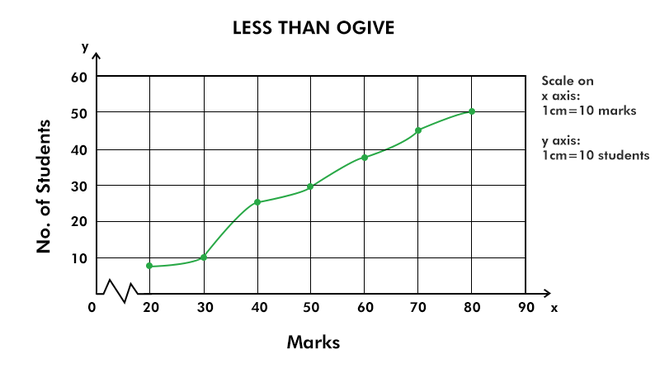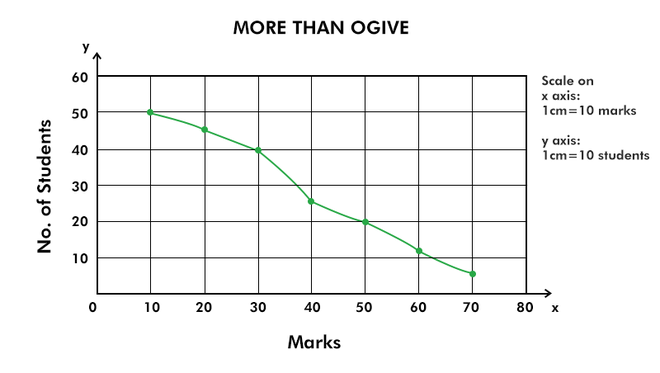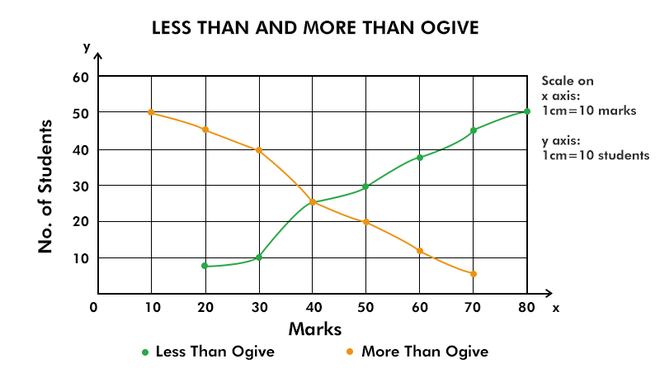GFG App
Open AppBrowser
Continue

# Ogive (Cumulative Frequency Curve) and its Types

A method of presenting data in the form of graphs that provides a quick and easier way to understand the trends of the given set of data is known as Graphic Presentation. A given set of data can be presented through the diagrammatic presentation; however, from the statistical point of view, graphic presentation is preferred by the investigators as it provides a more accurate and appropriate presentation of data. The graphic presentation of data facilitates the process of comparison of two or more situations. The two types of graphs through which a given set of data can be presented are Frequency Distribution Graphs and Time Series Graphs. The four most common graphs under Frequency Distribution Graphs are Line Frequency Graph, Histogram, Frequency Polygon, Frequency Curve, and Ogive.

## Ogive

Ogive or Cumulative Frequency Curve is a curve of a data set obtained by an individual through the representation of cumulative frequency distribution on a graph. As there are two types of cumulative frequency distribution; i.e., Less than cumulative frequencies and More than cumulative frequencies, the ogives are also of two types:

## Less than Ogive

The steps required to present a less than ogive graph are as follows:

#### Step 1

To present a less than ogive graph, add the frequencies of all the preceding class intervals to the frequency of a class.

#### Step 2

After that, plot the less than cumulative frequencies on the Y-axis against the upper limit of the corresponding class interval on the X-axis.

#### Step 3

In the last step, join these points by a smooth freehand curve, which is the resulting less than ogive.

A less than ogive curve is an increasing curve that slopes upwards from left to right.

#### Example:

Draw a ‘less than’ ogive curve from the following distribution of the marks of 50 students in a class.

#### Solution:

First of all, we have to convert the frequency distribution into a less than cumulative frequency distribution.

Now, plot these values of cumulative frequency on a graph.## More than Ogive

The steps required to present a more than ogive graph are as follows:

#### Step 1

To present a more than ogive graph, add the frequencies of all the succeeding class intervals to the frequency of a class.

#### Step 2

After that, plot the more than cumulative frequencies on the Y-axis against the upper limit of the corresponding class interval on the X-axis.

#### Step 3

In the last step, join these points by a smooth freehand curve, which is the resulting more than ogive.

A more than ogive curve is a decreasing curve that slopes downwards from left to right.

#### Example:

Draw a ‘more than’ ogive curve from the following distribution of the marks of 50 students in a class.

#### Solution:

First of all, we have to convert the frequency distribution into a more than cumulative frequency distribution.

Now, plot these values of cumulative frequency on a graph.## Both ‘Less Than’ and ‘More Than’ Ogives

Both the ‘less than’ and the ‘more than’ ogives can be plotted on the same graph, and the point at which these two curves intersect is the median of the given data set.

#### Example:

Draw both ‘less than’ and ‘more than’ ogive curve from the following distribution of the marks of 50 students in a class.

#### Solution:

First of all, we have to convert the frequency distribution into a less than and more than cumulative frequency distribution.

Now, plot these values of less than and more than cumulative frequency on a graph.My Personal Notes arrow_drop_up